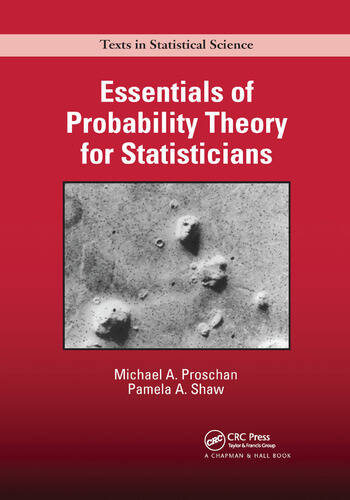## essentials of probability theory for statisticians

Posted in Books, Kids, pictures, Statistics, Travel, University life with tags , , , , , , , , , , , , on April 25, 2020 by xi'anOn yet another confined sunny lazy Sunday morning, I read through Proschan and Shaw’s Essentials of Probability Theory for Statisticians, a CRC Press book that was sent to me quite a while ago for review. The book was indeed published in 2016. Before moving to serious things, let me evacuate the customary issue with the cover. I have trouble getting the point of the “face on Mars” being adopted as the cover of a book on probability theory (rather than a book on, say, pareidolia). There is a brief paragraph on post-facto probability calculations, stating how meaningless the question of the probability of this shade appearing on a Viking Orbiter picture by “chance”, but this is so marginal I would have preferred any other figure from the book!

The book plans to cover the probability essentials for dealing with graduate level statistics and in particular convergence, conditioning, and paradoxes following from using non-rigorous approaches to probability. A range that completely fits my own prerequisite for statistics students in my classes and that of course involves the recourse to (Lebesgue) measure theory. And a goal that I find both commendable and comforting as my past experience with exchange students led me to the feeling that rigorous probability theory was mostly scrapped from graduate programs. While the book is not extremely formal, it provides a proper motivation for the essential need of measure theory to handle the complexities of statistical analysis and in particular of asymptotics. It thus relies as much as possible on examples that stem from or relate to statistics, even though most examples may appear as standard to senior readers. For instance the consistency of the sample median or a weak version of the Glivenko-Cantelli theorem. The final chapter is dedicated to applications (in the probabilist’ sense!) that emerged from statistical problems. I felt these final chapters were somewhat stretched compared with what they could have been, as for instance with the multiple motivations of the conditional expectation, but this simply makes for more material. If I had to teach this material to students, I would certainly rely on the book! in particular because of the repeated appearances of the quincunx for motivating non-Normal limites. (A typo near Fatou’s lemma missed the dominating measure. And I did not notice the Riemann notation dx being extended to the measure in a formal manner.)

[Disclaimer about potential self-plagiarism: this post or an edited version will eventually appear in my Books Review section in CHANCE.]

## sampling-importance-resampling is not equivalent to exact sampling [triste SIR]

Posted in Books, Kids, Statistics, University life with tags , , , , , , on December 16, 2019 by xi'anFollowing an X validated question on the topic, I reassessed a previous impression I had that sampling-importance-resampling (SIR) is equivalent to direct sampling for a given sample size. (As suggested in the above fit between a N(2,½) target and a N(0,1) proposal.)  Indeed, when one produces a sample$x_1,\ldots,x_n \stackrel{\text{i.i.d.}}{\sim} g(x)$

and resamples with replacement from this sample using the importance weights$f(x_1)g(x_1)^{-1},\ldots,f(x_n)g(x_n)^{-1}$

the resulting sample$y_1,\ldots,y_n$

is neither “i.” nor “i.d.” since the resampling step involves a self-normalisation of the weights and hence a global bias in the evaluation of expectations. In particular, if the importance function g is a poor choice for the target f, meaning that the exploration of the whole support is imperfect, if possible (when both supports are equal), a given sample may well fail to reproduce the properties of an iid example ,as shown in the graph below where a Normal density is used for g while f is a Student t⁵ density:## asymptotics of M³C²L

Posted in Statistics with tags , , , , , , , on August 19, 2018 by xi'anIn a recent arXival, Blazej Miasojedow, Wojciech Niemiro and Wojciech Rejchel establish the convergence of a maximum likelihood estimator based on an MCMC approximation of the likelihood function. As in intractable normalising constants. The main result in the paper is a Central Limit theorem for the M³C²L estimator that incorporates an additional asymptotic variance term for the Monte Carlo error. Where both the sample size n and the number m of simulations go to infinity. Independently so. However, I do not fully perceive the relevance of using an MCMC chain to target an importance function [which is used in the approximation of the normalising constant or otherwise for the intractable likelihood], relative to picking an importance function h(.) that can be directly simulated.Aware of my fascination for paradoxes (and heterodox publications), Ewan Cameron sent me the link to a recent arXival by Louis Lyons (Oxford) on different asymptotic distributions of the likelihood ratio. Which is full of approximations. The overall point of the note is hard to fathom… Unless it simply plans to illustrate Betteridge’s law of headlines, as suggested by Ewan.Tomorrow noon, I will give a talk at Trinity College Dublin on the asymptotic properties of ABC. (Here are the slides from the talk I gave in Berlin last month.)